﻿ How to calculate the PH of Benzoic Acid solution?
• 日本語
•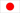• Português
•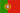• Française
•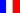• 简体中文
•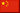• العربية
•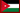• Español
•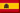• Русский
•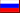• English
•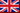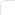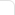## News

 How to calculate the PH of Benzoic Acid solution?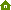Home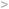NewsIndustry News How to calculate the PH of Benzoic Acid solution? Benzoic acid is a weak acid and it dissociates proton and benzoate ions after its reaction with water. benzoic acid dissociate equilibrium dissociate equilibrium Ka value Ka value benzoic acid pka and ph relationship between pka and ph Above is the dissociation equation of benzoic acid and the relationship between PKa and PH, HA stands for benzoic acid, A- stands for benzoate ion. Step 1: Suppose the concentration of total HA is 0.001 mol/L. There can be more benzoic acid soluble than 0.001 mol/L if at the temperature 25°C. To be exactly, the mol concentration would be n=solubility/molecular formula, that’s say n=3.44/122.123 = 0.028mol/L. Here using 0.001 mol/L just to make it easy. Step 2: Write the ionization equilibrium equation as follows: HA(aq)+H2O(l)⇌A−(aq)+H3O+(aq) [H3O+]  is the Hydronium concentration [A−] is the conjugate base concentration [HA] is the benzoic acid concentration The concentration of [A−] and [H3O+] is the same. And we suppose the concentration is X. And the final concentration of HA in solution is 0.001 – X. Step 3: Then the equation can be written X*X/0.001-X = Ka. As 0.001-X can be similar to 0.001, so we can get the X value 10-3.6, so the PH value is 3.6. Pre: Four types of Tartaric Acid Next: Crystalline Fructose VS High Fructose Corn Syrup?TEL: +86-21-50321522
+86-21-50321520
Fax:  +86-21-51069122Constantly strive towards:

★ High quality products
★ Professional support
★ Total solution for food
★ Reasonable price
★ Credible friendly cooperation

### Fooding Next Exhibitions:

Exhibition: Fi Europe & Ni 2019
Place: Paris, France
Time: 3 - 5 Dec, 2019
Booth No.: 7P39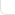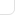﻿